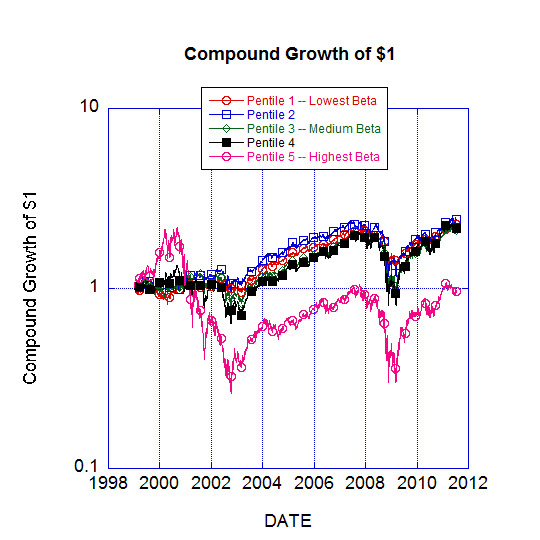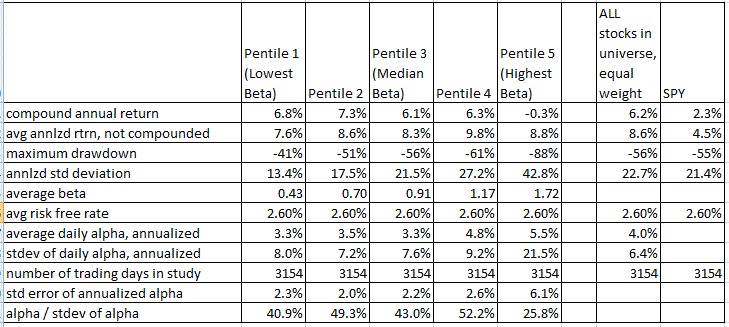# Risky vs. Non-Risky Stocks, from Charles Pennington

July 26, 2011 |

How has beta been working lately?

Attached is a graph of the compound growth since 1999 of \$1 dollar invested in each of five "pentiles" of S&P 500-like stocks, sorted according to each stock's trailing beta. (I will specify the technical details more precisely below.).

.

At first glance, pentile 5, the high beta stocks, looks like a disaster. After 12 years, it's at break-even, and that's after suffering enormous white-knuckle drawdowns of almost 90%. The other four pentiles all show an annualized compound returns of 6-8%, all with lower volatility.

So are high beta stocks a big disaster? Recently the idea has emerged that low risk stocks offer superior performance, or at least risk-adjusted performance, than high risk stocks. Eric Falkenstein wrote a great book (Finding Alpha ) and writes an ongoing blog on the broad theme that risky assets don't have the pay-off that they should, and recently some institutional offerings of low volatility stock portfolios have emerged, such as the Powershares Low Volatility S&P 500 ETF.

The results presented here seem at first to support the thesis that low risk stocks are the way to go, and that high risk stocks should be avoided. However, if you take a closer look, these results turn out to be in close agreement with the "Capital Asset Pricing Model" ("CAPM", the model of beta) and more broadly, the efficient market hypothesis.

Briefly, the reasons that high beta stocks have the illusion of underperforming are:

1) Because of their high volatility, their geometric compounded returns sharply lagged their arithmetic returns. That's not a fair comparison with low beta stocks, though, because an investor does not need to invest as many dollars in high beta stocks to get the equivalent market exposure. With a lower dollar exposure, the geometric and arithmetic returns would approach each other.

2) Because an investor doesn't need to invest as much money in high beta stocks to get the market return, he can use the remaining money to earn the risk-free rate — and that benefit is not properly credited to risky stocks in a simple plot of their compound returns.

Both of these issues can be assessed and addressed by analyzing data with the standard CAPM formula:

R - Rf = beta * ( Rspy - Rf) + alpha

where R = return, Rf is the risk-free rate, Rspy is the market return (which I take to be the return of the S&P 500 ETF SPY), and alpha is what efficient market purists would call an "error term", representing the amount by which the return was above or below what it "should" have been. From our point of view though, alpha is the out-performance or under-performance that we're trying to measure. Our question is, "Do high beta stocks have negative alpha?". The short answer is that they don't (or at least they didn't from 1999 to now).

Here is a summary spreadsheet of the statistics.The annualized alphas for pentiles 1 (low beta) to 5 (high beta) were 3.3%, 3.5%, 3.3%, 4.8%, and 5.5% respectively.

The reader may be very surprised that ALL the alphas are positive, which is sort of a "Lake Woebegone" effect. That's equivalent to the fact that the "equal weighted" S&P 500 index out-performed the traditional cap-weighted index over this period.

In any case, the alpha for pentile 5, the highest beta pentile, was 5.5%, the highest of any pentile. Pentile 1, the lowest beta pentile, was tied for the lowest alpha, at 3.3%. So from that point of view, the highest beta stocks were the best performers of all, despite having the worst compound annual return. To be fair, the annualized volatility of pentile 5's alpha was also the highest, and the ratio of pentile 5's alpha to the volatility of its alpha (which is equivalent to the Sharpe ratio of pentile 5, hedged with an appropriate short position in the S&P 500 index) was the lowest, so from that point of view pentile 5 was the worst performer (and pentile 4 the best).

We shouldn't over-analyze which of these alphas is the best, though, because within statistical error, they are all the same. The statistical error for the alphas of the pentiles are all about 2-3%, except for pentile 5, which has a 6% statistical error. The alphas themselves range from 3.3 to 5.5, and so the inescapable conclusion is that within statistical error ALL PENTILES WERE ABOUT THE SAME in terms of their alpha.

So my soapbox message is that the performance of high beta stocks may look terrible at first glance, but you've got to correct for the fact that you don't have to hold as much of them to get your market exposure. Don't penalize them by using geometric returns, and make sure to credit them for the interest you'd earn (or the margin interest that you wouldn't have to pay) because you don't have to hold as many dollars' worth of them.

TECHNICAL DETAILS:

1) This study used a database (MarketQA) that I believe to be free of survivorship bias

2) The 500 stocks used in the study are not the actual S&P 500
components. At the start of each calendar year, I selected the 500
largest market cap US domiciled stocks, trading on either NYSE or Nasdaq.

3) On each trading day the 500 stocks (excluding any that were trading
at less than \$5 per share as of the previous close) are sorted into 5
pentiles based on their total return beta with SPY. Beta is calculated for each stock based on the prior 250 trading days of close-to-close moves.

4) The daily return of each pentile was calculated as an equal-weight average of the daily returns of the (roughly) 100 stocks in each pentile.

5) The daily risk-free rate used is taken to be the 3-month treasury bill yield index (divided by 252, assuming 252 trading days per year). It averaged 2.6% over the period of the study, but it ranged from zero (currently) to values much greater than the average.

6) The daily alpha for each stock is calculated as alpha = (R-Rf) -beta * (Rspy - Rf). The alpha for each pentile is calculated as the equal weight average of the alphas of the (roughly) 100 stocks in the pentile.

7) "Annualized" alphas for each pentile is 252 times the average of the daily alphas. There were 3,154 trading days. Annualized standard deviations of the daily alphas of each pentile is the square root of 252 multiplied by the standard deviations of the daily alphas of each pentile.

# Comments

WordPress database error: [Table './dailyspeculations_com_@002d_dailywordpress/wp_comments' is marked as crashed and last (automatic?) repair failed]
`SELECT * FROM wp_comments WHERE comment_post_ID = '6607' AND comment_approved = '1' ORDER BY comment_date`

Name

Email

Website

Speak your mind